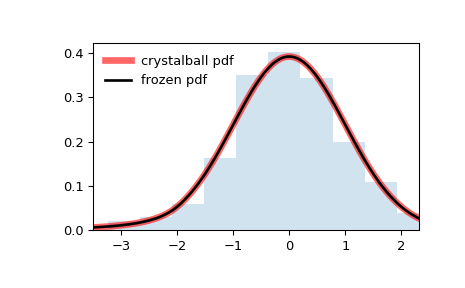# scipy.stats.crystalball¶

scipy.stats.crystalball = <scipy.stats._continuous_distns.crystalball_gen object>[source]

Crystalball distribution

As an instance of the rv_continuous class, crystalball object inherits from it a collection of generic methods (see below for the full list), and completes them with details specific for this particular distribution.

Notes

The probability density function for crystalball is:

$\begin{split}f(x, \beta, m) = \begin{cases} N \exp(-x^2 / 2), &\text{for } x > -\beta\\ N A (B - x)^{-m} &\text{for } x \le -\beta \end{cases}\end{split}$

where $$A = (m / |\beta|)^m \exp(-\beta^2 / 2)$$, $$B = m/|\beta| - |\beta|$$ and $$N$$ is a normalisation constant.

crystalball takes $$\beta > 0$$ and $$m > 1$$ as shape parameters. $$\beta$$ defines the point where the pdf changes from a power-law to a Gaussian distribution. $$m$$ is the power of the power-law tail.

References

1

“Crystal Ball Function”, https://en.wikipedia.org/wiki/Crystal_Ball_function

The probability density above is defined in the “standardized” form. To shift and/or scale the distribution use the loc and scale parameters. Specifically, crystalball.pdf(x, beta, m, loc, scale) is identically equivalent to crystalball.pdf(y, beta, m) / scale with y = (x - loc) / scale. Note that shifting the location of a distribution does not make it a “noncentral” distribution; noncentral generalizations of some distributions are available in separate classes.

New in version 0.19.0.

Examples

>>> from scipy.stats import crystalball
>>> import matplotlib.pyplot as plt
>>> fig, ax = plt.subplots(1, 1)


Calculate the first four moments:

>>> beta, m = 2, 3
>>> mean, var, skew, kurt = crystalball.stats(beta, m, moments='mvsk')


Display the probability density function (pdf):

>>> x = np.linspace(crystalball.ppf(0.01, beta, m),
...                 crystalball.ppf(0.99, beta, m), 100)
>>> ax.plot(x, crystalball.pdf(x, beta, m),
...        'r-', lw=5, alpha=0.6, label='crystalball pdf')


Alternatively, the distribution object can be called (as a function) to fix the shape, location and scale parameters. This returns a “frozen” RV object holding the given parameters fixed.

Freeze the distribution and display the frozen pdf:

>>> rv = crystalball(beta, m)
>>> ax.plot(x, rv.pdf(x), 'k-', lw=2, label='frozen pdf')


Check accuracy of cdf and ppf:

>>> vals = crystalball.ppf([0.001, 0.5, 0.999], beta, m)
>>> np.allclose([0.001, 0.5, 0.999], crystalball.cdf(vals, beta, m))
True


Generate random numbers:

>>> r = crystalball.rvs(beta, m, size=1000)


And compare the histogram:

>>> ax.hist(r, density=True, histtype='stepfilled', alpha=0.2)
>>> ax.legend(loc='best', frameon=False)
>>> plt.show()Methods

 rvs(beta, m, loc=0, scale=1, size=1, random_state=None) Random variates. pdf(x, beta, m, loc=0, scale=1) Probability density function. logpdf(x, beta, m, loc=0, scale=1) Log of the probability density function. cdf(x, beta, m, loc=0, scale=1) Cumulative distribution function. logcdf(x, beta, m, loc=0, scale=1) Log of the cumulative distribution function. sf(x, beta, m, loc=0, scale=1) Survival function (also defined as 1 - cdf, but sf is sometimes more accurate). logsf(x, beta, m, loc=0, scale=1) Log of the survival function. ppf(q, beta, m, loc=0, scale=1) Percent point function (inverse of cdf — percentiles). isf(q, beta, m, loc=0, scale=1) Inverse survival function (inverse of sf). moment(n, beta, m, loc=0, scale=1) Non-central moment of order n stats(beta, m, loc=0, scale=1, moments=’mv’) Mean(‘m’), variance(‘v’), skew(‘s’), and/or kurtosis(‘k’). entropy(beta, m, loc=0, scale=1) (Differential) entropy of the RV. fit(data) Parameter estimates for generic data. See scipy.stats.rv_continuous.fit for detailed documentation of the keyword arguments. expect(func, args=(beta, m), loc=0, scale=1, lb=None, ub=None, conditional=False, **kwds) Expected value of a function (of one argument) with respect to the distribution. median(beta, m, loc=0, scale=1) Median of the distribution. mean(beta, m, loc=0, scale=1) Mean of the distribution. var(beta, m, loc=0, scale=1) Variance of the distribution. std(beta, m, loc=0, scale=1) Standard deviation of the distribution. interval(alpha, beta, m, loc=0, scale=1) Endpoints of the range that contains fraction alpha [0, 1] of the distribution